• +91 9971497814
• info@interviewmaterial.com

# RD Chapter 13- Complex Numbers Ex-13.2 Interview Questions Answers

### Related Subjects

Question 1 :

Express the following complex numbers in the standard form a + ib:

(i) (1 + i) (1 + 2i)

(ii) (3 + 2i) / (-2 + i)

(iii) 1/(2 + i)2

(iv) (1 – i) / (1 + i)

(v) (2 + i)3 / (2 + 3i)

(vi) [(1 + i) (1 +√3i)] / (1 – i)

(vii) (2 + 3i) / (4 + 5i)

(viii) (1 – i)3 / (1 – i3)

(ix) (1 + 2i)-3

(x) (3 – 4i) / [(4 – 2i) (1 + i)]

(xi)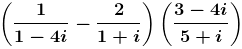(xii) (5 +√2i) / (1-√2i)

(i) (1 + i) (1 + 2i)

Let us simplify andexpress in the standard form of (a + ib),

(1 + i) (1 + 2i) =(1+i)(1+2i)

= 1(1+2i)+i(1+2i)

= 1+2i+i+2i2

= 1+3i+2(-1) [since, i=-1]

= 1+3i-2

= -1+3i

The values of a,b are -1, 3.

(ii) (3 + 2i) / (-2 + i)

Let us simplify andexpress in the standard form of (a + ib),

(3 + 2i) / (-2 + i) =[(3 + 2i) / (-2 + i)] × (-2-i) / (-2-i) [multiply and divide with (-2-i)]

= [3(-2-i) + 2i(-2-i)] / [(-2)2 – (i)2]

= [-6 -3i – 4i -2i2]/ (4-i2)

= [-6 -7i -2(-1)] / (4– (-1)) [since, i= -1]

= [-4 -7i] / 5

The values of a,b are -4/5, -7/5

(iii) 1/(2 + i)2

Let us simplify andexpress in the standard form of (a + ib),

1/(2 + i)2 =1/(22 + i2 + 2(2) (i))

= 1/ (4 – 1 + 4i)[since, i= -1]

= 1/(3 + 4i) [multiplyand divide with (3 – 4i)]

= 1/(3 + 4i) × (3 –4i)/ (3 – 4i)]

= (3-4i)/ (32 –(4i)2)

= (3-4i)/ (9 – 16i2)

= (3-4i)/ (9 – 16(-1))[since, i= -1]

= (3-4i)/25

The values of a,b are 3/25, -4/25

(iv) (1 – i) / (1 + i)

Let us simplify andexpress in the standard form of (a + ib),

(1 – i) / (1 + i) = (1– i) / (1 + i) × (1-i)/(1-i) [multiply and divide with (1-i)]

= (12 +i2 – 2(1)(i)) / (12 – i2)

= (1 + (-1) -2i) / (1– (-1))

= -2i/2

= -i

The values of a,b are 0, -1

(v) (2 + i)3 /(2 + 3i)

Let us simplify andexpress in the standard form of (a + ib),

(2 + i)3 /(2 + 3i) = (23 + i3 + 3(2)2(i) +3(i)2(2)) / (2 + 3i)

= (8 + (i2.i)+ 3(4)(i) + 6i2) / (2 + 3i)

= (8 + (-1)i + 12i +6(-1)) / (2 + 3i)

= (2 + 11i) / (2 + 3i)

[multiplyand divide with (2-3i)]

= (2 + 11i)/(2 + 3i) ×(2-3i)/(2-3i)

= [2(2-3i) +11i(2-3i)] / (22 – (3i)2)

= (4 – 6i + 22i – 33i2)/ (4 – 9i2)

= (4 + 16i – 33(-1)) /(4 – 9(-1)) [since, i= -1]

= (37 + 16i) / 13

The values of a,b are 37/13, 16/13

(vi) [(1 + i) (1 +√3i)] /(1 – i)

Let us simplify andexpress in the standard form of (a + ib),

[(1 +i) (1 +√3i)] / (1 – i) = [1(1+√3i) + i(1+√3i)] / (1-i)

= (1 + √3i + i + √3i2)/ (1 – i)

= (1 + (√3+1)i +√3(-1)) / (1-i) [since, i= -1]

= [(1-√3) + (1+√3)i] /(1-i)

[multiplyand divide with (1+i)]

= [(1-√3) + (1+√3)i] /(1-i) × (1+i)/(1+i)

= [(1-√3) (1+i) +(1+√3)i(1+i)] / (12 – i2)

= [1-√3+ (1-√3)i +(1+√3)i + (1+√3)i2] / (1-(-1)) [since, i= -1]

= [(1-√3)+(1-√3+1+√3)i+(1+√3)(-1)]/ 2

= (-2√3 + 2i) / 2

= -√3 + i

The values of a,b are -√3, 1

(vii) (2 + 3i) / (4 + 5i)

Let us simplify andexpress in the standard form of (a + ib),

(2 + 3i) / (4 + 5i) =[multiply and divide with (4-5i)]

= (2 + 3i) / (4 + 5i)× (4-5i)/(4-5i)

= [2(4-5i) + 3i(4-5i)]/ (42 – (5i)2)

= [8 – 10i + 12i – 15i2]/ (16 – 25i2)

= [8+2i-15(-1)] / (16– 25(-1)) [since, i= -1]

= (23 + 2i) / 41

The values of a,b are 23/41, 2/41

(viii) (1 – i)3 /(1 – i3)

Let us simplify andexpress in the standard form of (a + ib),

(1 – i)3 /(1 – i3) = [13 – 3(1)2i + 3(1)(i)2 –i3] / (1-i2.i)

= [1 – 3i + 3(-1)-i2.i]/ (1 – (-1)i) [since, i= -1]

= [-2 – 3i – (-1)i] /(1+i)

= [-2-4i] / (1+i)

[Multiplyand divide with (1-i)]

= [-2-4i] / (1+i) ×(1-i)/(1-i)

= [-2(1-i)-4i(1-i)] /(12 – i2)

= [-2+2i-4i+4i2]/ (1 – (-1))

= [-2-2i+4(-1)] /2

= (-6-2i)/2

= -3 – i

The values of a,b are -3, -1

(ix) (1 + 2i)-3

Let us simplify andexpress in the standard form of (a + ib),

(1 + 2i)-3 =1/(1 + 2i)3

= 1/(13+3(1)2 (2i)+2(1)(2i)2 +(2i)3)

= 1/(1+6i+4i2+8i3)

= 1/(1+6i+4(-1)+8i2.i)[since, i= -1]

= 1/(-3+6i+8(-1)i)[since, i= -1]

= 1/(-3-2i)

= -1/(3+2i)

[Multiplyand divide with (3-2i)]

= -1/(3+2i) ×(3-2i)/(3-2i)

= (-3+2i)/(32 –(2i)2)

= (-3+2i) / (9-4i2)

= (-3+2i) / (9-4(-1))

= (-3+2i) /13

The values of a,b are -3/13, 2/13

(x) (3 – 4i) / [(4 – 2i)(1 + i)]

Let us simplify andexpress in the standard form of (a + ib),

(3 – 4i) / [(4 – 2i)(1 + i)] = (3-4i)/ [4(1+i)-2i(1+i)]

= (3-4i)/ [4+4i-2i-2i2]

= (3-4i)/ [4+2i-2(-1)][since, i= -1]

= (3-4i)/ (6+2i)

[Multiplyand divide with (6-2i)]

= (3-4i)/ (6+2i) ×(6-2i)/(6-2i)

= [3(6-2i)-4i(6-2i)] /(62 – (2i)2)

= [18 – 6i – 24i + 8i2]/ (36 – 4i2)

= [18 – 30i + 8 (-1)]/ (36 – 4 (-1)) [since, i= -1]

= [10-30i] / 40

= (1 – 3i) / 4

The values of a,b are 1/4, -3/4

(xi)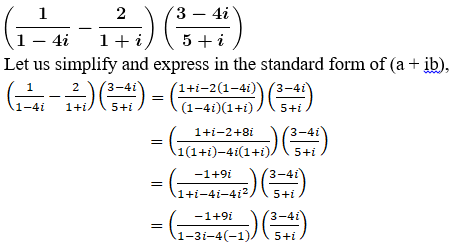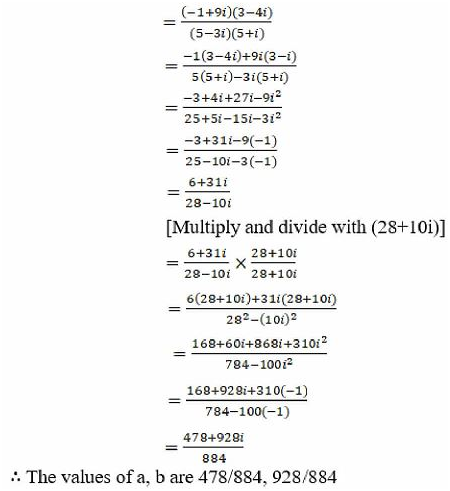(xii) (5 +√2i) / (1-√2i)

Let us simplify andexpress in the standard form of (a + ib),

(5 +√2i) / (1-√2i) =[Multiply and divide with (1+√2i)]

= (5 +√2i) / (1-√2i) ×(1+√2i)/(1+√2i)

= [5(1+√2i) + √2i(1+√2i)] / (12 –(√2)2)

= [5+5√2i + √2i + 2i2] / (1 – 2i2)

= [5 + 6√2i + 2(-1)] / (1-2(-1)) [since, i=-1]

= [3+6√2i]/3

= 1+ 2√2i

The values of a,b are 1, 2√2

Question 2 :

Find the real values of x and y, if

(i) (x + iy) (2 – 3i) = 4 + i

(ii) (3x – 2i y) (2 + i)2 = 10(1 + i)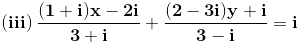(iv) (1 + i) (x + iy) = 2 – 5i

(i) (x + iy) (2 – 3i) = 4+ i

Given:

(x + iy) (2 – 3i) = 4+ i

Let us simplify theexpression we get,

x(2 – 3i) + iy(2 – 3i)= 4 + i

2x – 3xi + 2yi – 3yi=4 + i

2x + (-3x+2y)i – 3y(-1) = 4 + i [since, i= -1]

2x + (-3x+2y)i + 3y =4 + i [since, i= -1]

(2x+3y) + i(-3x+2y) =4 + i

Equating Real andImaginary parts on both sides, we get

2x+3y = 4… (i)

And -3x+2y = 1… (ii)

Multiply (i) by 3 and(ii) by 2 and add

On solving we get,

6x – 6x – 9y + 4y = 12+ 2

13y = 14

y = 14/13

Substitute the valueof y in (i) we get,

2x+3y = 4

2x + 3(14/13) = 4

2x = 4 – (42/13)

= (52-42)/13

2x = 10/13

x = 5/13

x = 5/13, y = 14/13

The real valuesof x and y are 5/13, 14/13

(ii) (3x – 2i y) (2 + i)2 =10(1 + i)

Given:

(3x – 2iy) (2+i)=10(1+i)

(3x – 2yi) (22+i2+2(2)(i))= 10+10i

(3x – 2yi) (4 +(-1)+4i) = 10+10i [since, i= -1]

(3x – 2yi) (3+4i) =10+10i

Let us divide with3+4i on both sides we get,

(3x – 2yi) =(10+10i)/(3+4i)

= Now multiply anddivide with (3-4i)

= [10(3-4i) +10i(3-4i)] / (32 – (4i)2)

= [30-40i+30i-40i2]/ (9 – 16i2)

= [30-10i-40(-1)] /(9-16(-1))

= [70-10i]/25

Now, equating Real andImaginary parts on both sides we get

3x = 70/25 and -2y =-10/25

x = 70/75 and y = 1/5

x = 14/15 and y = 1/5

The real valuesof x and y are 14/15, 1/5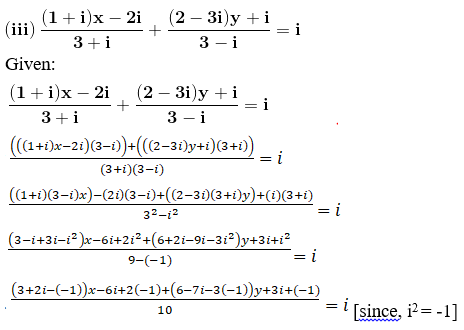(4+2i) x-3i-3 +(9-7i)y = 10i

(4x+9y-3) + i(2x-7y-3)= 10i

Now, equating Real andImaginary parts on both sides we get,

4x+9y-3 = 0 … (i)

And 2x-7y-3 = 10

2x-7y = 13 … (ii)

Multiply (i) by 7 and(ii) by 9 and add

On solving theseequations we get

28x + 18x + 63y – 63y= 117 + 21

46x = 117 + 21

46x = 138

x = 138/46

= 3

Substitute the valueof x in (i) we get,

4x+9y-3 = 0

9y = -9

y = -9/9

= -1

x = 3 and y = -1

The real valuesof x and y are 3 and -1

(iv) (1 + i) (x + iy) = 2 –5i

Given:

(1 + i) (x + iy) = 2 –5i

Divide with (1+i) onboth the sides we get,

(x + iy) = (2 –5i)/(1+i)

Multiply and divide by(1-i)

= (2 – 5i)/(1+i) ×(1-i)/(1-i)

= [2(1-i) – 5i (1-i)]/ (12 – i2)

= [2 – 7i + 5(-1)] / 2[since, i= -1]

= (-3-7i)/2

Now, equating Real andImaginary parts on both sides we get

x = -3/2 and y = -7/2

Thee real valuesof x and y are -3/2, -7/2

Question 3 :

Find the conjugates of the following complex numbers:

(i) 4 – 5i

(ii) 1 / (3 + 5i)

(iii) 1 / (1 + i)

(iv) (3 – i)2 / (2 + i)

(v) [(1 + i) (2 + i)] / (3 + i)

(vi) [(3 – 2i) (2 + 3i)] / [(1 + 2i) (2 – i)]

(i) 4 – 5i

Given:

4 – 5i

We know the conjugateof a complex number (a + ib) is (a – ib)

So,

The conjugate of (4 –5i) is (4 + 5i)

(ii) 1 / (3 + 5i)

Given:

1 / (3 + 5i)

Since the givencomplex number is not in the standard form of (a + ib)

Let us convert tostandard form by multiplying and dividing with (3 – 5i)

We get,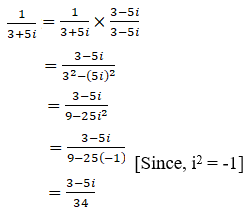We know the conjugateof a complex number (a + ib) is (a – ib)

So,

The conjugate of (3 –5i)/34 is (3 + 5i)/34

(iii) 1 / (1 + i)

Given:

1 / (1 + i)

Since the givencomplex number is not in the standard form of (a + ib)

Let us convert tostandard form by multiplying and dividing with (1 – i)

We get,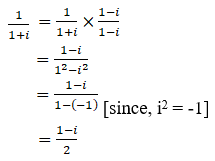We know the conjugateof a complex number (a + ib) is (a – ib)

So,

The conjugate of(1-i)/2 is (1+i)/2

(iv) (3 – i)2 /(2 + i)

Given:

(3 – i)2 /(2 + i)

Since the givencomplex number is not in the standard form of (a + ib)

Let us convert tostandard form,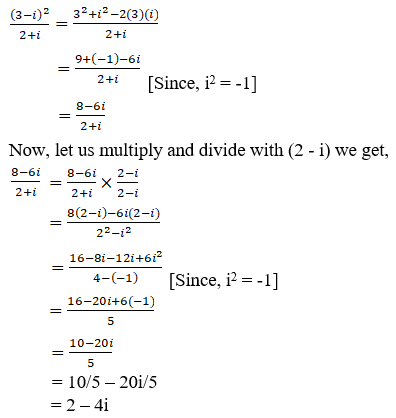We know the conjugateof a complex number (a + ib) is (a – ib)

So,

The conjugate of (2 –4i) is (2 + 4i)

(v) [(1 + i) (2 + i)] / (3+ i)

Given:

[(1 +i) (2 + i)] / (3 + i)

Since the givencomplex number is not in the standard form of (a + ib)

Let us convert tostandard form,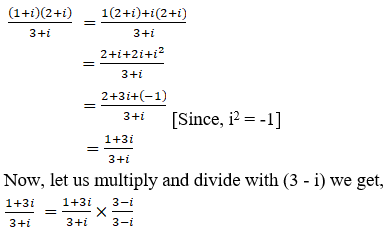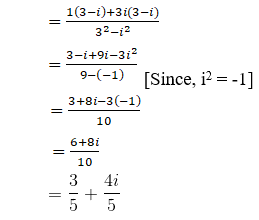We know the conjugateof a complex number (a + ib) is (a – ib)

So,

The conjugate of (3 +4i)/5 is (3 – 4i)/5

(vi) [(3 – 2i) (2 + 3i)] /[(1 + 2i) (2 – i)]

Given:

[(3 –2i) (2 + 3i)] / [(1 + 2i) (2 – i)]

Since the givencomplex number is not in the standard form of (a + ib)

Let us convert tostandard form,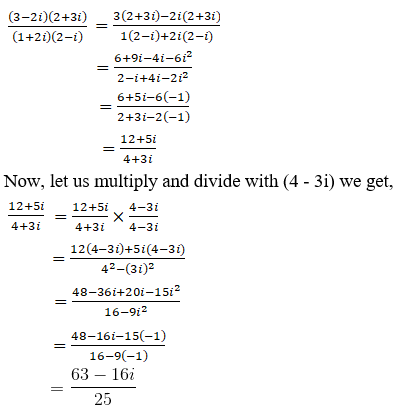We know the conjugateof a complex number (a + ib) is (a – ib)

So,

The conjugate of (63– 16i)/25 is (63 + 16i)/25

Question 4 :

Find the multiplicative inverse of the following complex numbers:

(i) 1 – i

(ii) (1 + i √3)2

(iii) 4 – 3i

(iv) √5 + 3i

(i) 1 – i

Given:

1 – i

We know themultiplicative inverse of a complex number (Z) is Z-1 or 1/Z

So,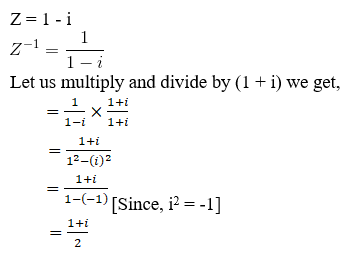The multiplicativeinverse of (1 – i) is (1 + i)/2

(ii) (1 + i √3)2

Given:

(1 + i √3)2

Z = (1 + i √3)2

= 12 +(i √3)2 + 2 (1) (i√3)

= 1 + 3i2 +2 i√3

= 1 + 3(-1) + 2 i√3[since, i2 = -1]

= 1 – 3 + 2 i√3

= -2 + 2 i√3

We know themultiplicative inverse of a complex number (Z) is Z-1 or 1/Z

So,

Z = -2 + 2 i√3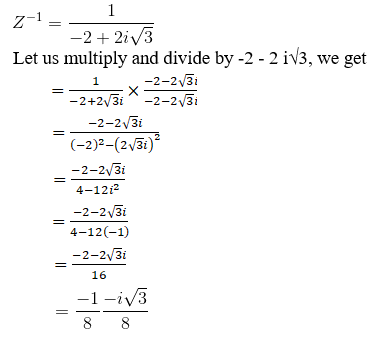The multiplicativeinverse of (1 + i√3)2 is (-1-i√3)/8

(iii) 4 – 3i

Given:

4 – 3i

We know themultiplicative inverse of a complex number (Z) is Z-1 or 1/Z

So,

Z = 4 – 3i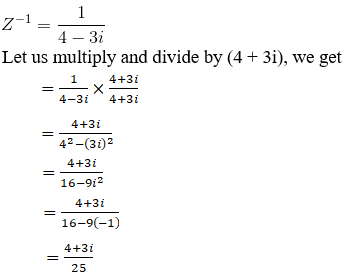The multiplicativeinverse of (4 – 3i) is (4 + 3i)/25

(iv) √5 + 3i

Given:

√5 + 3i

We know themultiplicative inverse of a complex number (Z) is Z-1 or 1/Z

So,

Z = √5 + 3i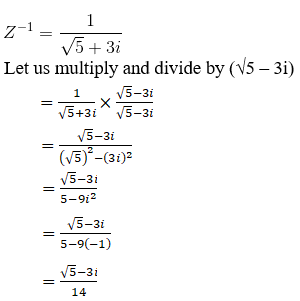The multiplicativeinverse of (√5 + 3i) is (√5 – 3i)/14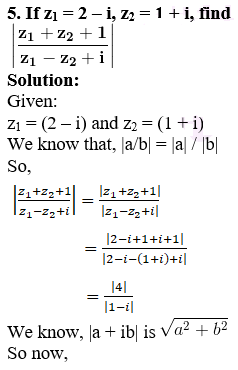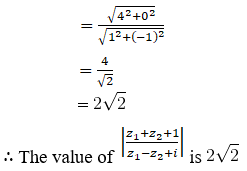Question 5 :

If z1 = (2 – i), z2 = (-2 + i), find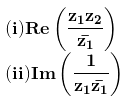Given:

z1 =(2 – i) and z2 = (-2 + i)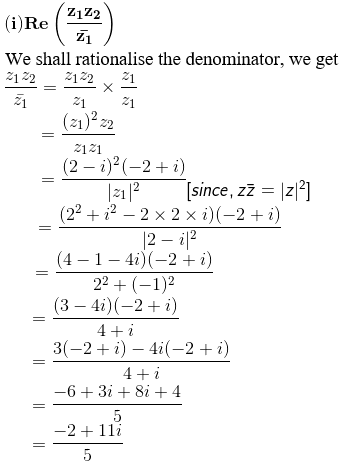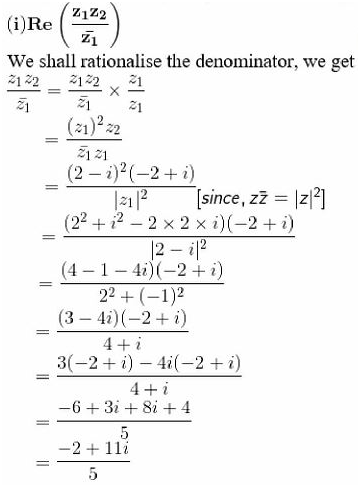Question 6 :

Find the modulus of [(1 + i)/(1 – i)] – [(1 – i)/(1 + i)]

Given:

[(1 +i)/(1 – i)] – [(1 – i)/(1 + i)]

So,

Z = [(1 + i)/(1 – i)]– [(1 – i)/(1 + i)]

Let us simplify, weget

= [(1+i) (1+i) – (1-i)(1-i)] / (12 – i2)

= [12 +i2 + 2(1)(i) – (12 + i2 –2(1)(i))] / (1 – (-1)) [Since, i2 = -1]

= 4i/2

= 2i

We know that for acomplex number Z = (a+ib) it’s magnitude is given by |z| = √(a2 + b2)

So,

|Z| = √(02 + 22)

= 2

The modulus of [(1 +i)/(1 – i)] – [(1 – i)/(1 + i)] is 2.

Question 7 :

If x + iy = (a+ib)/(a-ib), prove that x2 + y2 =1